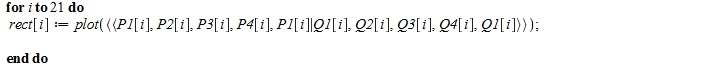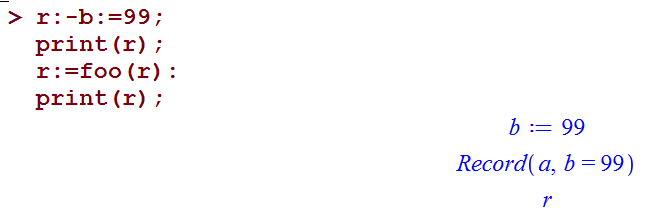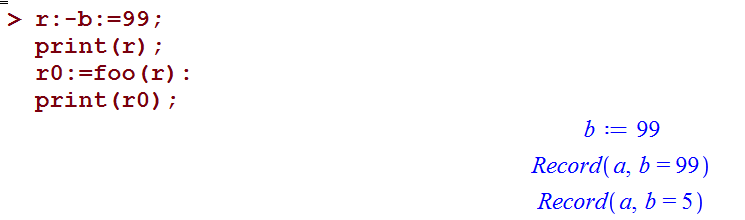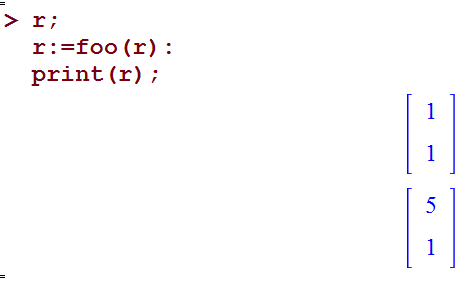## Adams-Bashforth method for second order ODE...

I want to solve an ODE of second order with Adams-Bashforth method.

It seems the solution has convergence for a small range of requested interval.

Thank you for taking your time

https://ufile.io/f9a0e

## Why Maple2018.1 cannot give the desired result for...

When I input the the following command line in Maple 2018.1

> Int(1/(1+sqrt(x)),x=0..1)=int(1/(1+sqrt(x)),x=0..1);

the output is

>Int(1/(1+sqrt(x)), x = 0 .. 1) = MeijerG([[0, 0, 1/2], []], [[1/2, 0], [-1]], 1)/Pi

which is not the desired result!  How can I get the desired result like this

>2-ln(2)

Thanks a lot.

## invalid subscript selector...

Goodday everyone,

Please, anyone with useful informations on the above stated error code should help.

Below is the attached file.

Error_invalid_subscript_selector.mw

Thanks in anticipation for your response

## Add a number to column values...

Please how do I add a number to column values in a matrix.

I needed to covert a column data representing temperatue values from celcius  to kelvin and I needed to add 273 to this column.

Thank you in advance.

## Real roots of a polynomial....

What is a good way to generate a random, high degree polynomial with integer coefficients, that has a high number of real roots. Example a degree 800 with 600 real roots. I am not looking for a mul application.

## How to get a real number output. ...

I entered this function
f:=x->(4*x^2-4)^(4/5);

Then I entered f(0) and got (-4)^(4/5).

When I tried to approximate , I got -2.452480922+1.781831689*I

I am looking for the real number value, which is 3.031433 with my TI-83 calculator and here https://www.desmos.com/calculator/b1soxuhbri

How do I set it in maple so that x is assumed to be real in f(x) and all outputs are real.

## How do I combine separate multiple rectangular int...

Hello everyone. Below is the loop coding to plot the rectangles.This will give me  21 rectangles with 21 graphs. And how am I going to combine them(21 rectangles) in one single graph? Really appreciate your help. Thank you in advanced! :)

## Function evaluation goes wrong, why?...

Hey and Thanks for your input.

 >(1)
 >Am I doing something wrong?

## How to enter complex numbers into Maple TA...

I am trying to create a question that generates two complex numbers and asks for the product of the two complex numbers.

This is what I have so far in the algorithm box of the question creator but it doesn't give me the correct answer:

\$a=5 + 20*I;
\$b=4 - 12*I;
\$ans= maple("evalc(\$a*\$b)");

This is my first time using MapleTA. Any help would be appreciated.

## 3D plot of explicit expression...

Hi

I am having trouble in plotting the 3d plot of explicit expressions. Lets say

eq1:=f(z)

eq2:=g(z)

then how can I plot f(z) and g(z) together with z in 3d plot?

Regards

Sunit

## Groebner Basis for System of Inequalities...

Can I create a Groebner basis in Maple with system of inequalities and how should I do that?

For example: I have problem with 30 inequlities (all have to be binary {0,1}) and all inequalities are >=1 but if I put

F := [x1+x2+x3-1, x1+x5+x7-1 etc]; and after that I create Groebner basis, I get that system has no solutions, but I know it has.

Thank you in advance!

## how did Maple obtain this solution to this ODE?...

in this ODE, with initial conditions, I am trying to verify my solution, but I can't figure how Maple obtained the final answer.  I can obtain same solution, but before using the initial conditions to find the constant of integrations. The problem comes in solving for the constant of integration from initial conditions. I do not know how Maple did it, becuause when I do the normal steps, I get division by zero.

```restart;
ode:=diff(y(x),x)=x*ln(y(x)):
ic:=y(1)=1:
sol:=dsolve({ode,ic},y(x));
```

gives "sol := y(x) = 1"

Now I solve without IC, then try to find _C1 by hand

```sol:=dsolve(ode,y(x));

sol := y(x) = exp(RootOf(x^2+2*Ei(1, -_Z)+2*_C1))
```

Now used remove_RootOf

```sol2:=DEtools:-remove_RootOf(sol);

sol2 := x^2+2*Ei(1, -ln(y(x)))+2*_C1 = 0
```

Now I followed the steps I learned at school, which is to plugin y=1 and x=1 in the solution to get an equation to solve for _C1

```eq := subs({y(x)=1,x=1},sol2);

eq := 1+2*Ei(1, -ln(1))+2*_C1 = 0
```

But Ei(1, -ln(1)) is a division by zero. So can't solve for _C1

```solve(eq,_C1);
Error, (in Ei) numeric exception: division by zero
```

So how would you solve for _C1 in the above? Or how did the smart Maple do it?

I tried using limits, but that did not help.  I tried using allvalues instead of remove_RootOf, and that did not get rid of RootOf.  Next I tried not to remove RootOf and keep it there to see what happens

```sol:=dsolve(ode,y(x));
eq := subs({y(x)=1,x=1},sol);
eq := 1 = exp(RootOf(1+2*Ei(1, -_Z)+2*_C1))

c1:=solve(eq,_C1, AllSolutions);
c1 := -1/2-Ei(1, -(2*I)*Pi*_Z1)

subs(_C1=c1,sol);
y(x) = exp(RootOf(x^2+2*Ei(1, -_Z)-1-2*Ei(1, -(2*I)*Pi*_Z1)))

simplify(%);
x^2+2*Ei(1, -ln(y(x)))-1-2*Ei(1, -(2*I)*Pi*_Z1) = 0

DEtools:-remove_RootOf(%);
x^2+2*Ei(1, -ln(y(x)))-1-2*Ei(1, -(2*I)*Pi*_Z1) = 0

solve(%,y(x));
exp(RootOf(x^2+2*Ei(1, -_Z)-1-2*Ei(1, -(2*I)*Pi*_Z1)))```

So I can't figure how Maple obtained y(x)=1. Maple must have used different method to solve for _C1.

What would be the Maple commands to use to obtain y(x)=1, starting from

sol:=dsolve(ode,y(x));

and given that y(1)=1 ?

## Easy simplification...

In the calculation below, A and B both simplify to 1.  Why doesn't A*B simplify to 1?  Tested on Maple 2017 and 2018.

 > restart;
 > A := (1 - cos(s)^2)/sin(s)^2;> B := (1 - cos(t)^2)/sin(t)^2;> simplify(A); simplify(B);Why doesn't this simplify to 1?

 > simplify(A*B);## another question on Record. overwritting Record on...

Can someone please explain in simple terms, why when I do

r:=foo(r), where "r" is a Record, then the returned  "r" is not what is returned from foo()?  I want to write a proc foo() which takes in a Record variable, update some of its fields, and then return the updated Record to the caller

```r:=Record('a','b');
foo:=proc(r)
r:-b:=5;
return(r);
end proc;```

But now when I call the above as follows

```r:-b:=99;
print(r);
r:=foo(r):
print(r);
```

The last print above just prints "r" and not the Record. It seems to have erased the Record.But it works, if I change the name of the variable to return the result into, as

```r:-b:=99;
print(r);
r0:=foo(r):
print(r0);
```When I do the same on say a Matrix, there is no problem

```restart;
r:=<<1,1>>:
foo:=proc(r)
r:=5;
return(r);
end proc;
```

And now

```r;
r:=foo(r):
print(r);
```Why it worked with a Matrix but not with Record? Why can't one overwrite the Record on the call return?

What would be the correct way to pass in a Record to a function, and have the function update some of its fields, and then return back the updated copy of the Record without having to make a new variable "r0" as above?